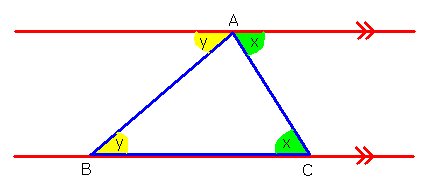# C Program To Check If Triangle Is Valid Or Not | C Programs

C Program to Input Angles of a triangle to check its validity – In this article, we will brief in on the only way to find out whether a triangle is valid or not after inputting its angles.

Suitable examples and sample programs have also been added so that you can understand the whole thing very clearly. The compiler has also been added with which you can execute it yourself.

As it is evident by the name of the title, firstly, you need to enter the angles of a triangle.

All the three angles of a triangle sum up to 180 degrees, no matter what.As you can see, in the triangle ABC, angle BAC, angle y and angle x constitute the angles.

The red lines are drawn parallel to each other. Thus, both the alternate interior angles are equal.

Since it is a line, as the name suggests, the whole of angle A is 180 degrees.

Thus, the sum of all the three angles is 180 degrees, making the triangle a valid one.

Hence, the only way to find out whether a triangle is valid or not in C programming is as follows:

## Using Standard Method

1)Read three angles of a triangle.

2)Calculate the sum of three angles of a triangle.

3)If the total sum of the three angles of a triangle is equal to 180 degrees and none of the angles is equal to zero, then the triangle is valid. Otherwise, print “triangle is invalid”.

Output:

x

## C Program To Check If Alphabet, Digit or Special Character | C Programs

C program to check for an alphabet, digit or special character – In this article, ...